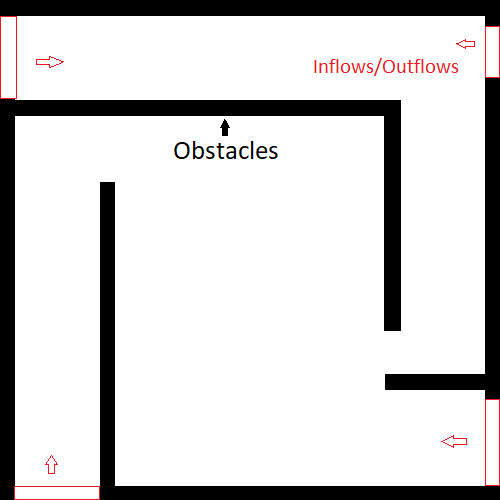# How to model multiple Inflow/Outflow fixed locations + parameterize inflow velocities?

Hi, I am trying to train a steady-state scenario with obstacles and multiple inflow/outflow ‘windows’ at fixed locations. The inflow velocities through these ‘windows’ are to be a parameter. At all times, at least one ‘window’ is outlet (pressure = 0 imposed). As a start. we can assume the inflow velocity through each of the other window(s) to be either 1 m/s or 0 m/s (although eventually the inlet velocities are to modelled as in range(0.0 ,1.0) with spacing of 0.2 m/s).

I have the following confusions:

How to add ‘inflow’/‘outflow’ constraints to the domain in this case? I am not sure if I have to consider an approach where inflow/outflow locations are also a parameter. If yes, could you please guide a way to create and use such a (discrete?) (vector?) parameter that tells which of the 4 ‘windows’ are inlets and outlets? The velocities through each of the inlet windows is another parameter.

Thank you for your excellent framework.The inclusion of multiple inlet/outflow boundaries should be fairly easy to achieve using multiple boundary constraints. I would suggest having a look at the Annular Ring example where there is a simple inlet and outlet boundary condition. I would personally start with learning a problem where the inlet/outlet locations are fixed and constant.

Such problems, where there are multiple constraints, is what the Modulus framework is designed for. Set up a geometry to represent the domain, then define your constraints using geometric criteria. As an example lets consider this domain is scaled to a unit square, your code may look something like this (the key here is the sympy criteria arguments):

``````# This code doesn't but serves as a starting point
geo = Rectangle((0,0), (1,1))

# Top left inlet with u = 1
inlet_1 = PointwiseBoundaryConstraint(
nodes=nodes,
geometry=geo,
outvar={"u": 1, "v": 0},
batch_size=cfg.batch_size.inlet,
criteria=And(Eq(x, 0), y > 0.9 ),
)

# Top right inlet with u = -1
inlet_2 = PointwiseBoundaryConstraint(
nodes=nodes,
geometry=geo,
outvar={"u": -1, "v": 0},
batch_size=cfg.batch_size.inlet,
criteria=And(Eq(x, 1), y > 0.9 ),
)

# Bottom left inlet with v = 1
inlet_3 = PointwiseBoundaryConstraint(
nodes=nodes,
geometry=geo,
outvar={"u": 0, "v": 1},
batch_size=cfg.batch_size.inlet,
criteria=And(Eq(y, 0), x < 0.1 ),
)